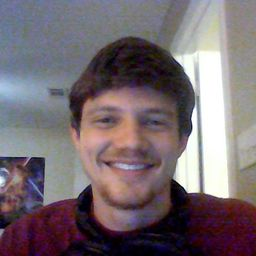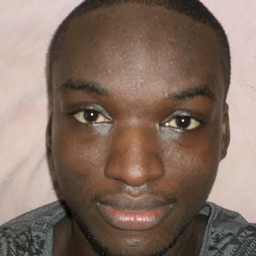## Educators

YZ
agCP

Problem 1

Suppose you start at the origin, move along the x-axis a distance of 4 units in the positive direction, and then move downward a distance of 3 units. What are the coordinates of your position?

YZ
Yiming Z.

Problem 2

Sketch the points $(1, 5, 3)$, $(0, 2, -3)$, $(-3, 0, 2)$, and $(2, -2, -1)$ on a single set of coordinate axes.

ag
Alan G.

Problem 3

Which of the points $A (-4, 0, -1)$, $B (3, 1, -5)$, and $C (2, 4, 6)$ is closest to the $yz$-plane? Which point lies in the $xz$-plane?

YZ
Yiming Z.

Problem 4

What are the projections of the point $(2, 3, 5)$ on the $xy$-, $yz$-, and $xz$- planes? Draw a rectangular box with the origin and $(2, 3, 5)$ as opposite vertices and with its faces parallel to the
coordinate planes. Label all vertices of the box. Find the length of the diagonal of the box.

Check back soon!

Problem 5

What does the equation $x = 4$ represent in $\mathbb{R}^2$? What does it represent in $\mathbb{R}^3$? Illustrate with sketches.

YZ
Yiming Z.

Problem 6

What does the equation $y = 3$ represent in $\mathbb{R}^3$? What does $z = 5$ represent? What does the pair of equations $y = 3$, $z = 5$ represent? In other words, describe the set of points $(x, y, z)$ such that $y = 3$ and $z = 5$. Illustrate with a sketch.

YZ
Yiming Z.

Problem 7

Describe and sketch the surface in $\mathbb{R}^3$ represented by the equation $x + y = 2$.

ag
Alan G.

Problem 8

Describe and sketch the surface $\mathbb{R}^3$ represented by the equation $x^2 + z^2 = 9$.

ag
Alan G.

Problem 9

Find the lengths of the sides of the triangle $PQR$. Is it a right triangle? Is it an isosceles triangle?

$P (3, -2, -3)$ , $Q (7, 0, 1)$ , $R (1, 2, 1)$

ag
Alan G.

Problem 10

Find the lengths of the sides of the triangle $PQR$. Is it a right triangle? Is it an isosceles triangle?

$P (2, -1, 0)$ , $Q (4, 1, 1)$ , $R (4, -5, 4)$

ag
Alan G.

Problem 11

Determine whether the points lie on a straight line.

(a) $A (2, 4, 2)$ , $B (3, 7, -2)$, $C (1, 3, 3)$
(b) $D (0, -5, 5)$ , $E (1, -2, 4)$, $F (3, 4, 2)$

Check back soon!

Problem 12

Find the distance from $(4, -2, 6)$ to each of the following.

(a) The $xy$-plane (b) The $yz$-plane
(c) The $xz$-plane (d) The $x$-axis
(e) The $y$-axis (f) The $z$-axisProblem 13

Find an equation of the sphere with center $(-3, 2, 5)$ and radius 4. What is the intersection of this sphere with the $yz$-plane?

ag
Alan G.

Problem 14

Find an equation of the sphere with center $(2, -6, 4)$ and radius 5. Describe its intersection with each of the coordinate planes.

ag
Alan G.

Problem 15

Find an equation of the sphere that passes through the point $(4, 3, -1)$ and has center $(3, 8, 1)$.

ag
Alan G.

Problem 16

Find an equation of the sphere that passes through the origin and whose center is $(1, 2, 3)$.

YZ
Yiming Z.

Problem 17

Show that the equation represents a sphere, and find its center and radius.

$x^2 + y^2 + z^2 - 2x - 4y + 8z = 15$

ag
Alan G.

Problem 18

Show that the equation represents a sphere, and find its center and radius.

$x^2 + y^2 + z^2 + 8x - 6y + 2z + 17 = 0$

ag
Alan G.

Problem 19

Show that the equation represents a sphere, and find its center and radius.

$2x^2 + 2y^2 + 2z^2 = 8x - 24z + 1$

ag
Alan G.

Problem 20

Show that the equation represents a sphere, and find its center and radius.

$3x^2 + 3y^2 + 3z^2 = 10 + 6y + 12z$

ag
Alan G.

Problem 21

(a) Prove that the midpoint of the line segment from $P_1 (x_1, y_1, z_1)$ to $P_2 (x_2, y_2, z_2)$ is
$$\left(\frac{x_1 + x_2}{2} , \frac{y_1+ y_2}{2} , \frac{z_1+ z_2}{2} \right)$$
(b) Find the lengths of the medians of the triangle with vertices $A (1, 2, 3)$, $B (-2, 0, 5)$, $C (4, 1, 5)$. (A median of a triangle is a line segment that joins a vertex to the midpoint of the opposite side.)

Check back soon!

Problem 22

Find an equation of a sphere if one of its diameters has endpoints $(5, 4, 3)$ and $(1, 6, -9)$.

YZ
Yiming Z.

Problem 23

Find equations of the spheres with center $(2, -3, 6)$ that touch (a) the $xy$-plane, (b) the $yz$-plane, (c) the $xz$-plane.

Check back soon!

Problem 24

Find an equation of the largest sphere with center $(5, 4, 9)$ that is contained in the first octant.

Check back soon!

Problem 25

Describe in words the region of $\mathbb{R}^3$ represented by the equation(s) or inequality.

$x = 5$

YZ
Yiming Z.

Problem 26

Describe in words the region of $\mathbb{R}^3$ represented by the equation(s) or inequality.

$y = -2$

ag
Alan G.

Problem 27

Describe in words the region of $\mathbb{R}^3$ represented by the equation(s) or inequality.

$y < 8$

ag
Alan G.

Problem 28

Describe in words the region of $\mathbb{R}^3$ represented by the equation(s) or inequality.

$z \ge -1$

ag
Alan G.

Problem 29

Describe in words the region of $\mathbb{R}^3$ represented by the equation(s) or inequality.

$0 \le z \le 6$

ag
Alan G.

Problem 30

Describe in words the region of $\mathbb{R}^3$ represented by the equation(s) or inequality.

$y^2 = 4$

ag
Alan G.

Problem 31

Describe in words the region of $\mathbb{R}^3$ represented by the equation(s) or inequality.

$x^2 + y^2 = 4$, $z = -1$

ag
Alan G.

Problem 32

Describe in words the region of $\mathbb{R}^3$ represented by the equation(s) or inequality.

$x^2 + y^2 = 4$

YZ
Yiming Z.

Problem 33

Describe in words the region of $\mathbb{R}^3$ represented by the equation(s) or inequality.

$x^2 + y^2 + z^2 = 4$

YZ
Yiming Z.

Problem 34

Describe in words the region of $\mathbb{R}^3$ represented by the equation(s) or inequality.

$x^2 + y^2 + z^2 \le 4$

ag
Alan G.

Problem 35

Describe in words the region of $\mathbb{R}^3$ represented by the equation(s) or inequality.

$1 \le x^2 + y^2 + z^2 \le 5$

ag
Alan G.

Problem 36

Describe in words the region of $\mathbb{R}^3$ represented by the equation(s) or inequality.

$x = z$

Check back soon!

Problem 37

Describe in words the region of $\mathbb{R}^3$ represented by the equation(s) or inequality.

$x^2 + z^2 \le 9$

Check back soon!

Problem 38

Describe in words the region of $\mathbb{R}^3$ represented by the equation(s) or inequality.

$x^2 + y^2 + z^2 > 2z$Isaac B.

Problem 39

Write inequalities to describe the region.
The region between the yz-plane and the vertical plane $x = 5$

Check back soon!

Problem 40

The solid cylinder that lies on or below the plane $z = 8$ and on or above the disk in the $xy$-plane with center the origin and radius 2.

Check back soon!

Problem 41

The region consisting of all points between (but not on) the spheres of radius $r$ and $R$ centered at the origin, where $r < R$.

CP
Connor P.

Problem 42

The solid upper hemisphere of the sphere of radius 2 centered at the origin

Check back soon!

Problem 43

The figure shows a line $L_1$ in space and a second line $L_2$, which is the projection of $L_1$ onto the $xy$-plane. (In other words, the points on $L_2$ are directly beneath, or above, the points on $L_1$.)
(a) Find the coordinates of the point $P$ on the line $L_1$.
(b) Locate on the diagram the points $A$, $B$, and $C$, where the line $L_1$ intersects the $xy$-plane, the $yz$-plane, and the $xz$-plane, respectively.

Check back soon!

Problem 44

Consider the points $P$ such that the distance from $P$ to $A (-1, 5, 3)$ is twice the distance from $P$ to $B (6, 2, -2)$. Show that the set of all such points is a sphere, and find its center and radius.

Check back soon!

Problem 45

Find an equation of the set of all points equidistant from the points $A (-1, 5, 3)$ and $B (6, 2, -2)$. Describe the set.

Check back soon!

Problem 46

Find the volume of the solid that lies inside both of the spheres
$$x^2 + y^2 + z^2 + 4x -2y + 4z + 5 = 0$$
and
$$x^2 + y^2 + z^2 = 4$$

Check back soon!

Problem 47

Find the distance between the spheres $x^2 + y^2 + z^2 = 4$ and $x^2 + y^2 + z^2 = 4x + 4y + 4z - 11$.

Check back soon!

Problem 48

Describe and sketch a solid with the following properties. When illuminated by rays parallel to the z-axis, its shadow is a circular disk. If the rays are parallel to the y-axis, its shadow is a square. If the rays are parallel to the x-axis, its shadow is an isosceles triangle.

Check back soon!